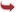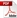International Journal of Scientific and Research Publications

#### IJSRP, Volume 5, Issue 3, March 2015 Edition [ISSN 2250-3153]An Econometric Model of Inflation in India
Dr.Debesh Bhowmik
Abstract: The paper verified that the inflation model in India is cointegrated in the order I(1) considering GDP growth rate, degree of openness, growth rate of money supply, nominal exchange rate of Rupee with respect to US\$ , fiscal deficit as per cent of GDP, interest rate (lending rate ), and crude oil price US\$/barrel respectively as the determinants during the study period of 1970-2013.Johansen cointegration test showed that there is one cointegrating vector as shown by trace statistics and there are two cointegrating vectors as shown by λmax statistics. VECM showed that the inflation rate is associated with interest rate in one period lag and previous period inflation rate is associated with GDP growth rate and growth of money supply significantly. VECM states that the estimated equation 1 is adjusting its error by 23%, equation 2 is adjusting by 14% equation 3 by 34% and equation 7 by 71% respectively within one year but others equations are adjusting negligibly. There are six AR polynomial unit roots and all others roots lie inside the unit circle which proves that the model is unstable where the impulse response functions are diverging.
[VIEW FULL PAPER][DOWNLOAD]

Reference this Research Paper (copy & paste below code):

Dr.Debesh Bhowmik (2018); An Econometric Model of Inflation in India; Int J Sci Res Publ 5(3) (ISSN: 2250-3153). http://www.ijsrp.org/research-paper-0315.php?rp=P393832